INTRODUCTION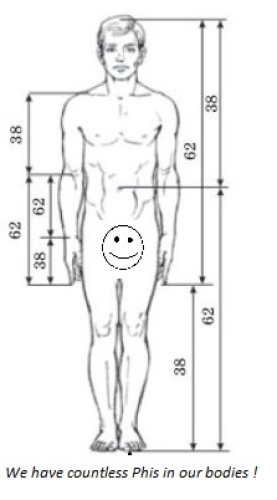Despite being technical analysis indicators, the powerful Fibonacci tools are widely used even by fundamental analysis traders. Unlike the common lagging indicators, they can predict future price levels . I leave the debate of whether this is a self-fulfilling prophecy or a bias toward a law in nature to others. Those tools will be explained in this article one by one.

FIBONACCI & THE FIBONACCI NUMBER SEQUENCE

Fibonacci was a thirteenth-century talented mathematician who described the numerical series now named after him. In the Fibonacci sequence of numbers, after 0 and 1, each number is the sum of the two prior numbers. Hence, the sequence is as follows: 0, 1, 1, 2, 3, 5, 8, 13, 21, 34, 55, 89, 144, 233, 377, 610 and so on extending to infinity. Each number is approximately 1.618 times greater than the preceding number.

THE GOLDEN RATIO

This figure 1.618 is called Phi or the Golden Ratio. The inverse of 1.618 is 0.618. The golden ratio appears everywhere in Nature from the micro scale to the macro scale. Things that are based on this divine proportion are beautiful and shapely and things that do not contain it look ugly and seem suspicious and unnatural.

FIBONACCI LEVELS USED IN THE FINANCIAL MARKETS

The financial markets also seem to conform to this golden ratio. In many cases, it is believed that humans subconsciously seek out the golden ratio. The levels used in Fibonacci tools are ratios derived from mathematical relationships between numbers in the sequence. The common Fibonacci levels are 23.6%,38.2%,50%,61.8%,76.4%,78.6%,100%,161.8%,261.8% and 423.6%.`

Every year new methods are developed for traders to take advantage of the uncanny tendencies of the market toward derivatives of the golden ratio.

1. FIBONACCI RETRACEMENTS

Fibonacci retracements are the most widely used of all the Fibonacci trading tools. They are levels at which the market is expected to retrace to after a strong trend, before resuming in its initial trend direction.

Steps to draw Fibonacci retracements

1. Find an ongoing trend. Identify the top and the bottom of the trend. This can be a highly subjective matter that depends on our own judgment.
2. Use a charting software or a platform, which has Fibonacci function; connect the bottom to the top (wick to wick)
3. The most common Fibonacci levels are 38.2%, 50% and 62.8%. The levels are customizable. On occasion, I find 23.6% and 78.6% usefulStrategies using Fibonacci retracements include :

1. A trader looking for an entry point into a market that has made a substantial move to the upside may place limit orders attempting to buy near the 38.2% or 50% retracement levels, with a stop-loss order placed either:
• Below the next Fibonacci level down (for example, an attempt to buy near the 50% level, with a stop below the 61.8% level).This requires perfect entry and more confidence that the support or resistance area will hold.
• Past the recent swing high or swing low (the 100% level). This is a litter safer, gives the trade more room to breathe, and gives a better chance for the market to move in favor. This can also lead to some unfavorable reward-to-risk ratios.
2. If a trader has recognized a market reversal following a substantial move up and accordingly entered a sell position, he or she may use the Fibonacci retracement levels as profit targets.

3. Significant violation of the 61.8% level is often considered an indication of complete market reversal of direction. If this happens, traders may enter short positions, expecting the market to move substantially lower.

Here comes the big question. Which retracement level to trade? Which one has the highest probability of market reversal?
The answer is simple. This Fibonacci tool, and all Fibonacci tools, can provide reliable trade setups, but not without confirmation. It is best used as a tool within a broader strategy that looks for the confluence of a number of indicators. The likelihood of a reversal increases if there is a confluence of technical signals when price reaches a Fibonacci level.
Other popular technical indicators that are used in conjunction with Fibonacci levels include candlestick patterns, trendlines, momentum oscillators and moving averages.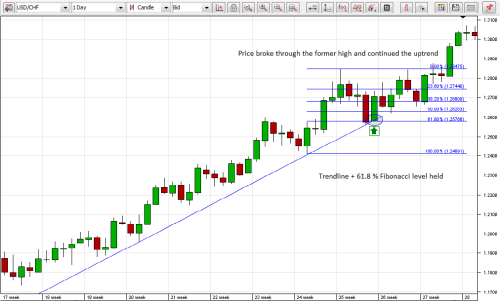Be aware that when applying a Fibonacci tool, the shorter your time frame, the less reliable the levels are.

2. FIBONACCI EXTENSIONS

Fibonacci retracement levels can be used to forecast potential areas of support or resistance at which traders can enter the market with a view to catching the resumption of an initial trend. Fibonacci extensions can complement this strategy by giving traders Fibonacci based profit targets.The major Fibonacci extension levels are 61.8%, 100%, 161.8%, 261.8% and 423.6%.
To draw Fibonacci Expansions we select the tool and click 3 times on the chart at the beginning of the primary move, the end of the primary move and the retracement.
Now back to the first USD/CHF example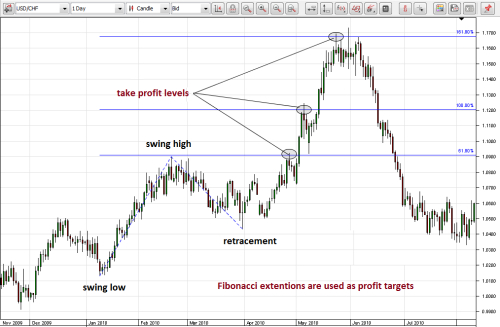Scaling out of a trade with multiple profit targets is an effective money management strategy allowing you to lock in profits as the position matures.

3. FIBONACCI CIRCLES (ARCS)

Fibonacci circles are an exciting way to use Fibonacci ratios, because they take into account both linear price measurements and time. Whereas the Fibonacci Retracements Tool shows static retracement levels, the Fibonacci Arcs show dynamic retracements that evolve over time.
Finding the high and low of a chart is the first step to composing Fibonacci arcs. Then, with a compass-like movement, three main curved lines are drawn at 38.2%, 50% and 61.8%, from the desired point. These lines anticipate the support and resistance levels, and areas of ranging.A popular strategy is to combine Fibonacci Arcs with Fibonacci fans and the points where the two indicators cross will indicate extremely strong areas of S/R.

4. FIBONACCI FANS

The exciting Fibonacci Fans use Fibonacci ratios based on time and price to construct support and resistance trendlines. After the high and low of the chart is located, Fibonacci fan lines are drawn as diagonal lines at 23.6%, 38.2%, 50% and 61.8% levels. The distance between fan lines becomes wider the further the trend extends.The lines create candidate support and resistance areas.Because fan lines are drawn to accommodate a trend, you do not “have” to move the anchor points until the trend moves outside, either above or below, the range of those lines.
Be aware that very steep trends are not very conducive to this kind of analysis .In these situations the market almost immediately moves outside the range of the Fibonacci fans and limits its usefulness. Alternatively, very fast trends or corrections can be ideal situations for a Fibonacci retracements to find potential areas of support or resistance.

5. FIBONACCI TIME ZONES

Unlike the other Fibonacci methods, time zones are a series of vertical lines that conform to the Fibonacci sequence (1, 1, 2, 3, 5, 8, 13, etc.). After locating the significant high and the significant low, they are composed to indicate areas in which major price movements can be expected. (I.e. lows or highs).After a downtrend, a reversal would be expected at a significant Fibonacci time extension line. Similarly, after an uptrend, a reversal warning could occur if a Fibonacci Time Extension was soon approaching .Fibonacci time forecasts work best when combined with either a retracement or fan study.

6. FIBONACCI CHANNELS

Fibonacci channels are less popular variants of Fibonacci retracement strategy, with support and resistance lines running diagonally rather than horizontally. A trader first draws a base channel. Then, additional parallel lines are drawn above the descending channel (resistance lines) or below the ascending channel (support lines) at levels .618 of the channel size, 1, 1.618, 2, 2.618, etc.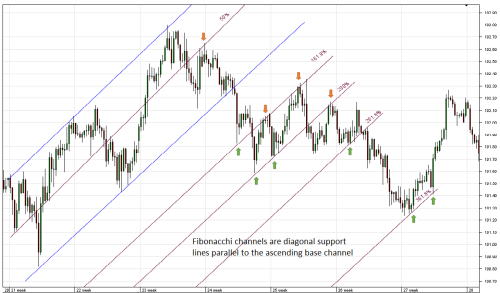Fibonacci channels are often drawn along with Fibonacci retracements. The points where the diagonal lines and horizontal lines cross are considered exceptionally strong levels of support or resistance for the market.

7. FIBONACCI CLUSTERS

Fibonacci clusters are an advanced tool for finding confluence of price retracements and extensions drawn from different highs and lows. The more Fibonacci levels are assigned to a cluster, the stronger the cluster is and the greater the likelihood that the price will reverse at this support or resistance level. Fibonacci Time Clusters work in the same way in identifying when a trend reversal may happen.Some indicators show these clusters on the chart Y-axis, beside the price, either as histograms or like series of horizontal bars with various degrees of shading. Each retracement level that overlaps with another makes the horizontal bar on the side darker at that price level. This is a great visual trading aid showing support and resistance.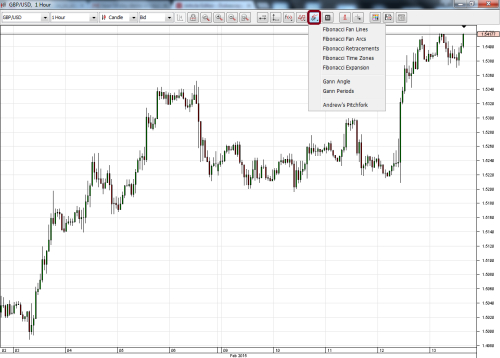Good luck Traders!